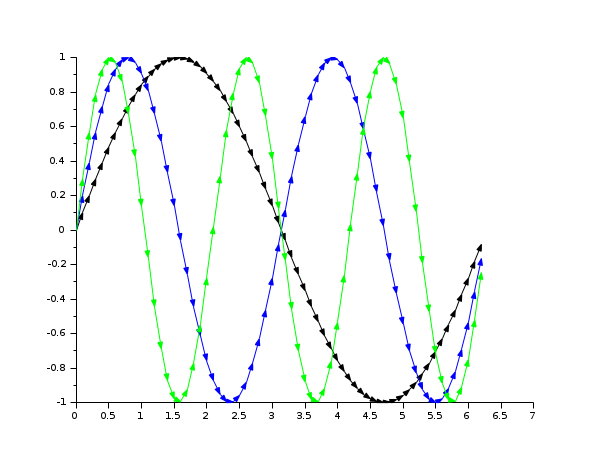Scilab Home page | Wiki | Bug tracker | Forge | Mailing list archives | ATOMS | File exchange
Change language to: Français - Português - 日本語 - Русский

See the recommended documentation of this function

Scilab Help >> Graphics > 2d_plot > plot2d4

# plot2d4

2D plot (arrows style)

### Calling Sequence

```plot2d4([logflag,] x,y,[style,strf,leg,rect,nax])
plot2d4(y)
plot2d4(x,y <,opt_args>)```

### Arguments

args

see `plot2d` for a description of parameters.

### Description

`plot2d4` is the same as `plot2d` but curves are plotted using arrows style. This can be useful when plotting solutions of an ODE in a phase space.

By default, successive plots are superposed. To clear the previous plot, use `clf()`.

Enter the command `plot2d4()` to see a demo.Note that all the modes proposed by `plot2dxx` (xx = 1 to 4) can be enabled using `plot2d` and setting the `polyline_style` option to the corresponding number.

### Sample### Examples

```// compare the following with plot2d1
x=[0:0.1:2*%pi]';
plot2d4(x,[sin(x) sin(2*x) sin(3*x)])
clf()
plot2d(x,[sin(x) sin(2*x) sin(3*x)])
e=gce();
e.children(1).polyline_style=4;
e.children(2).polyline_style=4;
e.children(3).polyline_style=4;```• fchamp — direction field of a 2D first order ODE
• plot2d — 2D plot
• plot2d2 — 2D plot (step function)
• plot2d3 — 2D plot (vertical bars)
• subplot — divide a graphics window into a matrix of sub-windows
• clf — Clear or reset or reset a figure or a frame uicontrol.
• polyline_properties — description of the Polyline entity properties